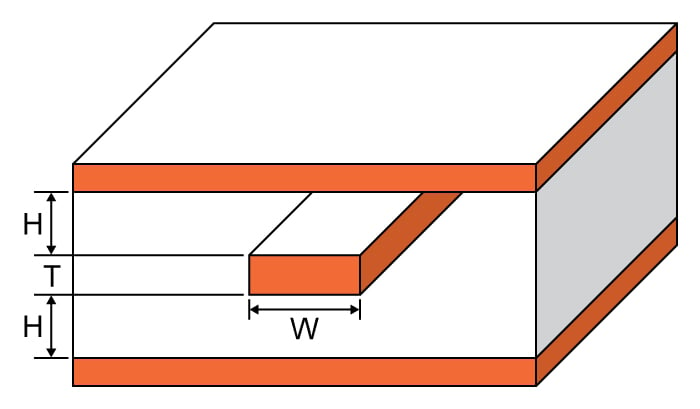# Stripline Max Current Calculator

## A calculator designed to calculate the maximum current that a stripline can handle

Amps
°C
Ohms
Volts
Watts

### Overview

This calculator is designed to compute the maximum allowable current that can flow through a stripline. To use this tool, enter the trace width, thickness, ambient temperature, temperature rise, and the microstrip length and click the "calculate" button.

A stripline is similar to the microstrip except that the flat conductor is now inside the dielectric material instead of on top of it. This serves the same purpose as the microstrip but is less likely to radiate signals because of its structure. The stripline can be used as an impedance for very high frequencies.### Equations

$$I_{max} = \sqrt{ \frac{\Delta T}{215.3W^{-1.15} T^{-1.0}}}$$

$$R = \frac{\rho_{cu} L}{A} (1+\alpha_{cu} \Delta T)$$

$$V = I_{max} R$$

$$P = V I_{max}$$

Where:

$$\Delta T$$ = temperature rise

$$W$$ = trace width

$$T$$ = trace thickness

$$L$$ = trace length

$$A$$ = trace cross-sectional area

$$\rho_{cu}$$ = resistivity of Copper = 1.7 x 10-6

$$\alpha_{cu}$$ = temperature coefficient of Copper = 3.9 x 10-3

*The maximum current formula is derived through curve fitting data found on IPC-2221

### Applications

A stripline is a very popular device in very high frequency circuits, particularly microwave circuits. It was invented along with the microstrip primarily to replace standard capacitors and inductors which are very difficult to manufacture when the frequency is very high. And just like the ordinary capacitors and inductors, striplines also have maximum ratings. One of the most important maximum ratings that engineers are concerned about is the maximum current which is what this calculator solves.•kubeek June 07, 2019
•RK37 June 13, 2019